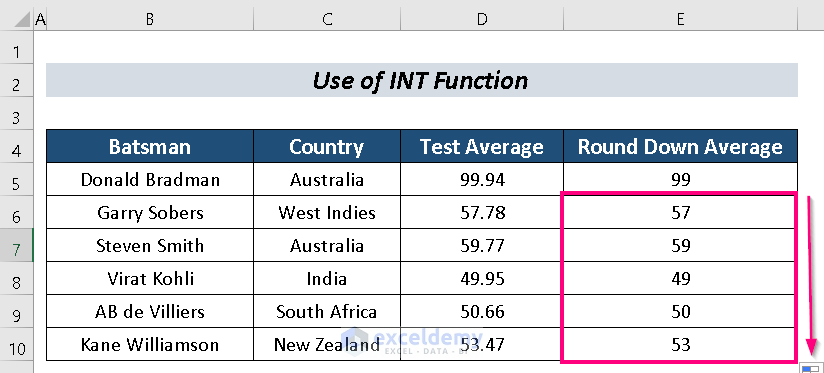# How to Round Down to Nearest Whole Number in Excel (4 Methods)

Get FREE Advanced Excel Exercises with Solutions!

We try to make our dataset both beautiful and simple at the same time.  In order to decorate our data, sometimes we face the necessity to round down to the nearest whole number in Excel. Here, I will try to discuss 4 smooth approaches to do that.

For more simplification, I am going to use a Dataset with the columns Batsman, Country, Test Average, and Round Down Average.## How to Round Down to Nearest Whole Number in Excel: 4 Smooth Approaches

### 1. Applying ROUNDDOWN Function

There is a function named ROUNDDOWN Function. We can easily round down to the nearest whole number in Excel with it.

Steps:

• Select a cell (e. D5).
• Input the following formula:
`=ROUNDDOWN(D5,0)`

Where,

D5 = The number we want to round down

0 = The number of digits to which we want in the decimal positions• Press ENTER.• Now, use Fill Handle to AutoFill the rests.Read More: How to Round Excel Data to Make Summations Correct

### 2. Using FLOOR Function to Round Down to Nearest Whole Number

The FLOOR Function is an amazing function to perform it perfectly.

Steps:

• Choose a cell (e. D5).
• Now, input the following formula:
`=FLOOR(D5,1)`

Where,

D5 = The number we want to round down

1 = The multiple to which we want to round the number• Hit ENTER.• AutoFill the remaining cells.### 3. Round Down to Nearest Whole Number Utilizing TRUNC Function

We can also use the TRUNC Function to perform our purpose.

Steps:

• Pick a cell (e. D5).
• Write the following formula in that cell:
`=TRUNC(D5,0)`

Where,

D5 = The number we want to round down

0 = The number of digits to which we want in the decimal positions• Next, press ENTER.• Finally, AutoFill the rests.Read More: How to Roundup a Formula Result in Excel

### 4. Using INT Function

Steps:

• First of all, select a cell. I selected cell D5.
• Next, input the following formula in the D5 cell:
`=INT(D5)`

Where,

D5 = The number that we want to round down• Press ENTER.• AutoFill to finish the process.

###Read More: Round off Formula in Excel Invoice

## Practice Section

You can practice here for more expertise.## Conclusion

In this article, I mentioned 4 Smooth approaches to round down to the nearest whole number in Excel. Hopefully, it will be helpful for Excel users. For any more questions, comment below.

## Related ArticlesNaimul Hasan Arif

Hello, I am Arif. I am an Engineer who graduated from BUET. I want to pursue an enterprising career in a progressive environment where my skills & knowledge can be enhanced to their maximum potential to contribute to the overall success and growth of the organization.

We will be happy to hear your thoughtsAdvanced Excel Exercises with Solutions PDF